Statistical analysis¶

For statistical analysis of the mechanical property values distribution, it is possible to use histograms or to use a cumulative distribution.

Probability density function (PDF)¶

Using histograms is really interesting for the visualization point of view with a common pattern, like the bell–shaped curve. Such a curve is known as the “normal distribution” or the “Gaussian distribution”. For an homogeneous material, only 1 peak is expected and for an heterogeneous material, a peak per phase can be expected. In this last case, intermediate peaks between phase peaks can be observed when interfaces are not spatially negligible. The drawback of this plot is the user-dependence of the bin size and thus of the distribution shape (i.e. peak intensity). Indeed, the shape of the distributions is bin size dependent, while this bin size (e.g. 0.5GPa or 3GPa) is defined arbitrary by the user. This issue is well known in the literature and is a little bit discussed in this presentation  and this paper . The shape of the distributions is also bin step dependent. In other words, if the the histogram starts from an odd or an even value, the distribution is different.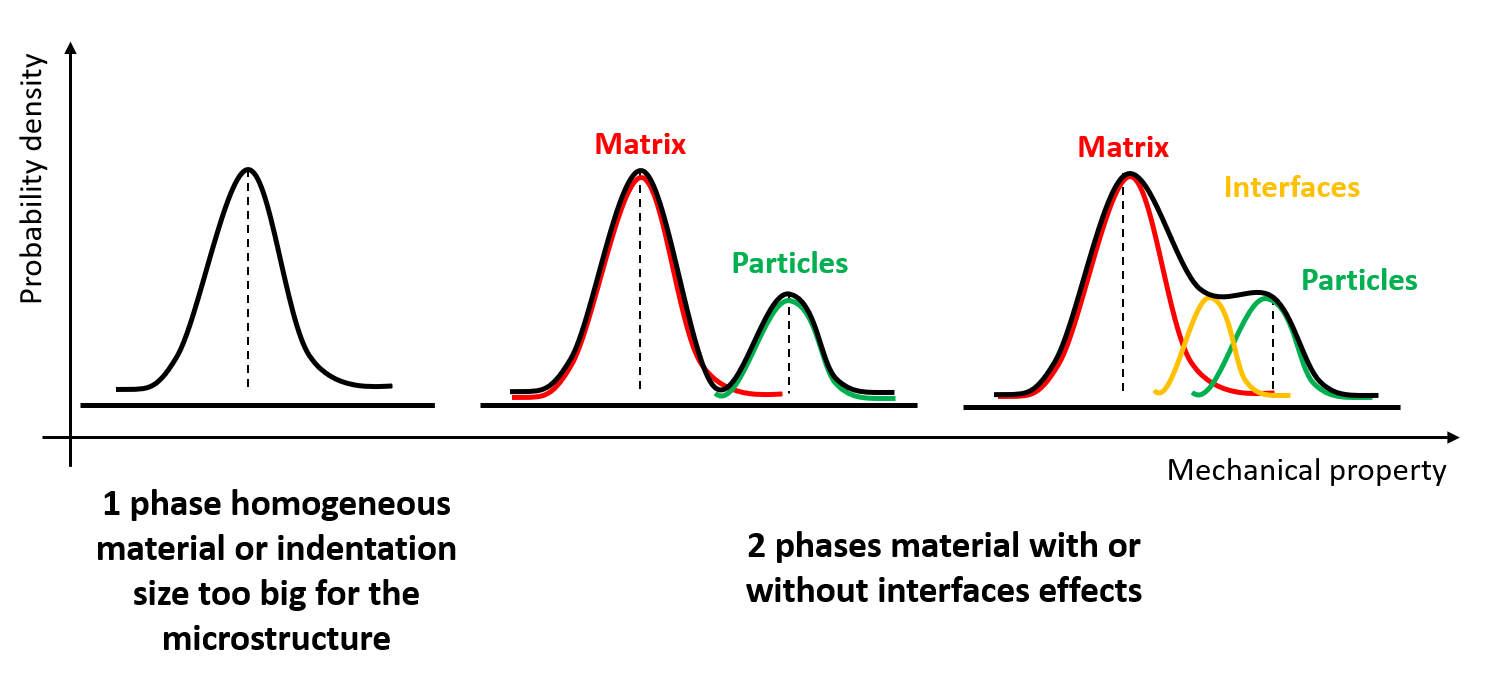Figure 23 Example of different fitted histogram distribution schematized as a function of the specimen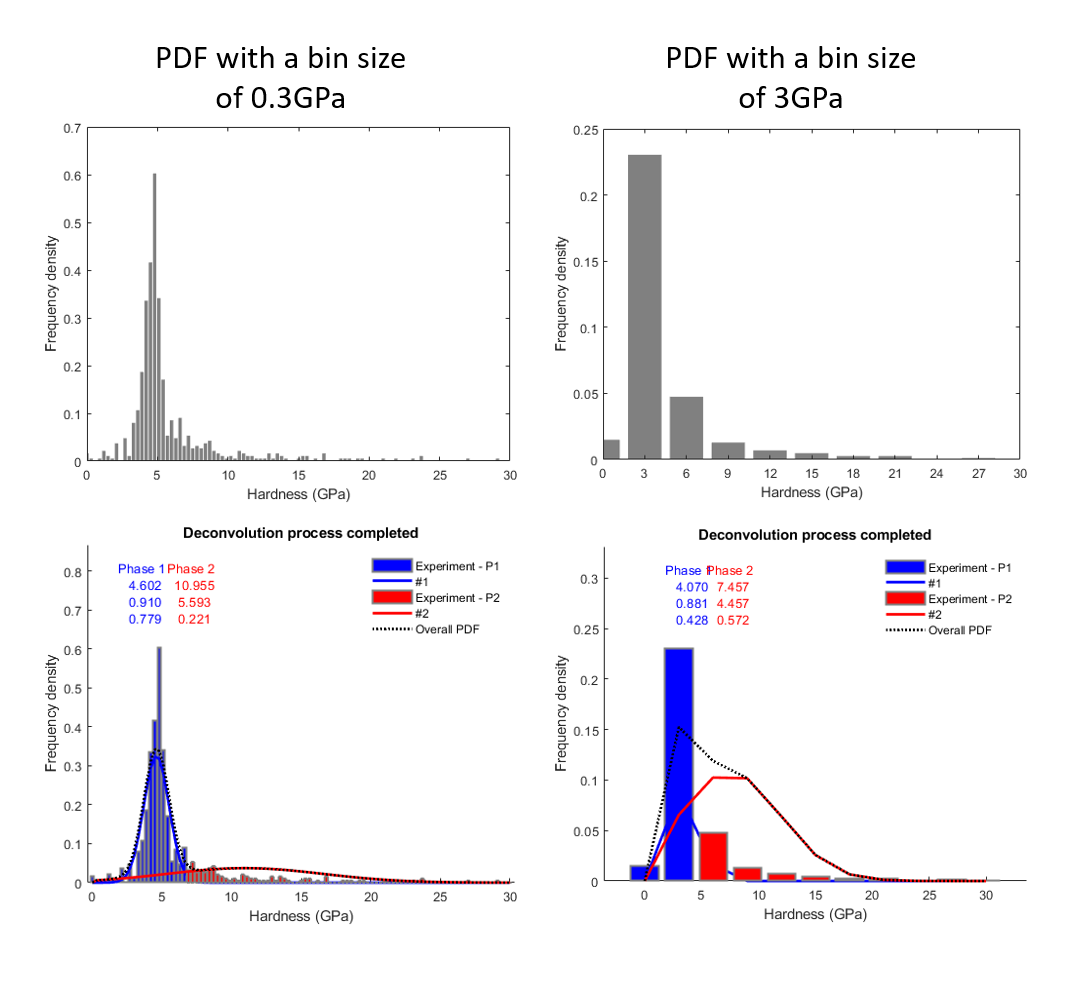Figure 24 Effect of the bin size definition on the distribution shape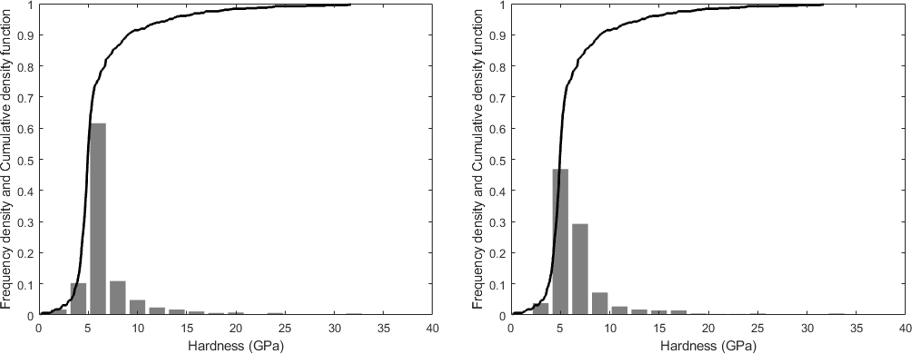Figure 25 Effect of the bin step on the distribution shape: the histogram start from 0GPa on the left and from 1GPa on the right, using the same bin size of 2GPa.

The next step is to fit this distribution using a probability density function. Such mathematical approach is very well defined in the literature ,  and . It is worth to note that the result obtain after deconvolution (average values and standard deviations for each peak) is dependent of the bin size. A solution to avoid user definition of the bin size is to use the Freedman–Diaconis rule of thumb, which gives an estimation of the bin size after calculation the interquartile (IQR) range of the data . To activate this option, check the box for ‘Auto Bin Size’ on the GUI.

(3)$\text{Bin Size} = \frac{2*IQR}{n^{\frac{1}{3}}}$

With $$n$$ is the number of observations in the sample.

The Matlab function used to calculate the interquartile range of the data is: iqrVal.m

Another rule of thumb is to use between 6 and 20 bins in a histogram.

The Matlab function used to plot the distribution of mechanical property values using histogram is: pdfGaussian.m

The Matlab function used to fit using a probability density function and to process the deconvolution is: TriDiMap_runDeconvolution.m

This last function has been extensively inspired by the work of Němeček J. et al. ,  and .

An example of fitting and deconvolution process is given in the following figure.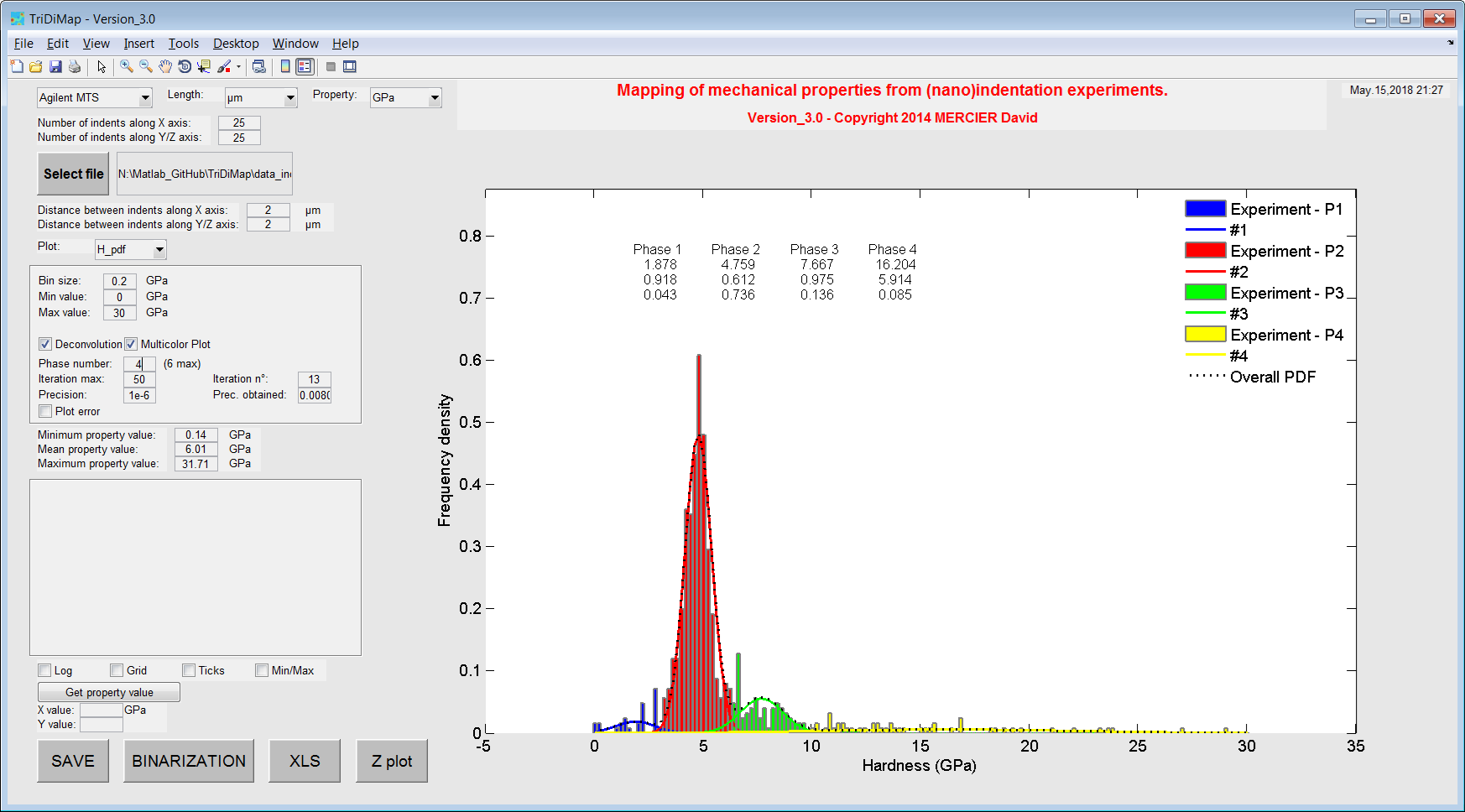Figure 26 Histograms of hardness values with Gaussian PDF after fitting and deconvolution step

Note

Effect of the interphase can be considered and could be implemented in this toolbox .

Note

The choice of the bin size could be defined as an automatic calculation, based on the number of phases, the number of data and the minimum of peak intensity…

When data are noisy due to experimental artefacts (e.g. surface contamination, interfaces effect or interesting phase inside the sample…) for example, with very high or very low mechanical property values, it is always possible to cut the signal, by setting manually (on the GUI) the extrema. This operation can be seen as an arbitrary cleaning, but careful with a fitting process, which gives different mean values, given peak shapes or peak number modification.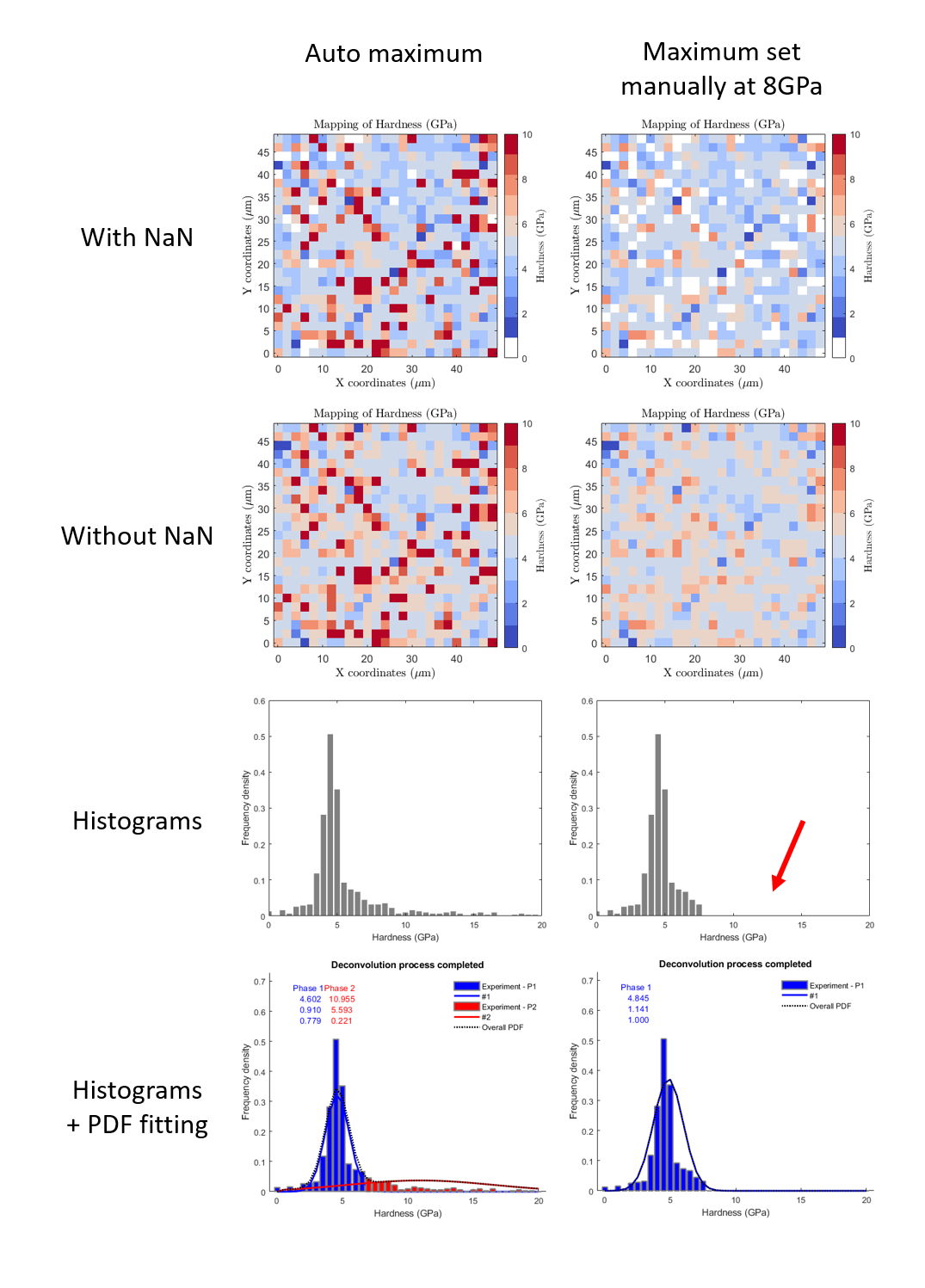Figure 27 Example of manually saturated indentation data, with a comparison between automatic maximum and a maximum set to 8GPa

Note

Sometimes the fit does not converge, just restart the fitting process… or change a little bit the bin size.

Cumulative density function (CDF)¶

The cumulative distribution of mechanical property is much better than an histogram plot. But, it is much more difficult to decompose and in this toolbox, only a Weibull fitting is proposed, which is only interesting for an homogeneous material. The Weibull function is from the PopIn toolbox .

The Matlab function used to plot the cumulative distribution of mechanical property values is: cdfGaussian.m

The Matlab function used to fit the cumulative distribution with a Weibull function is: TriDiMap_Weibull_cdf.m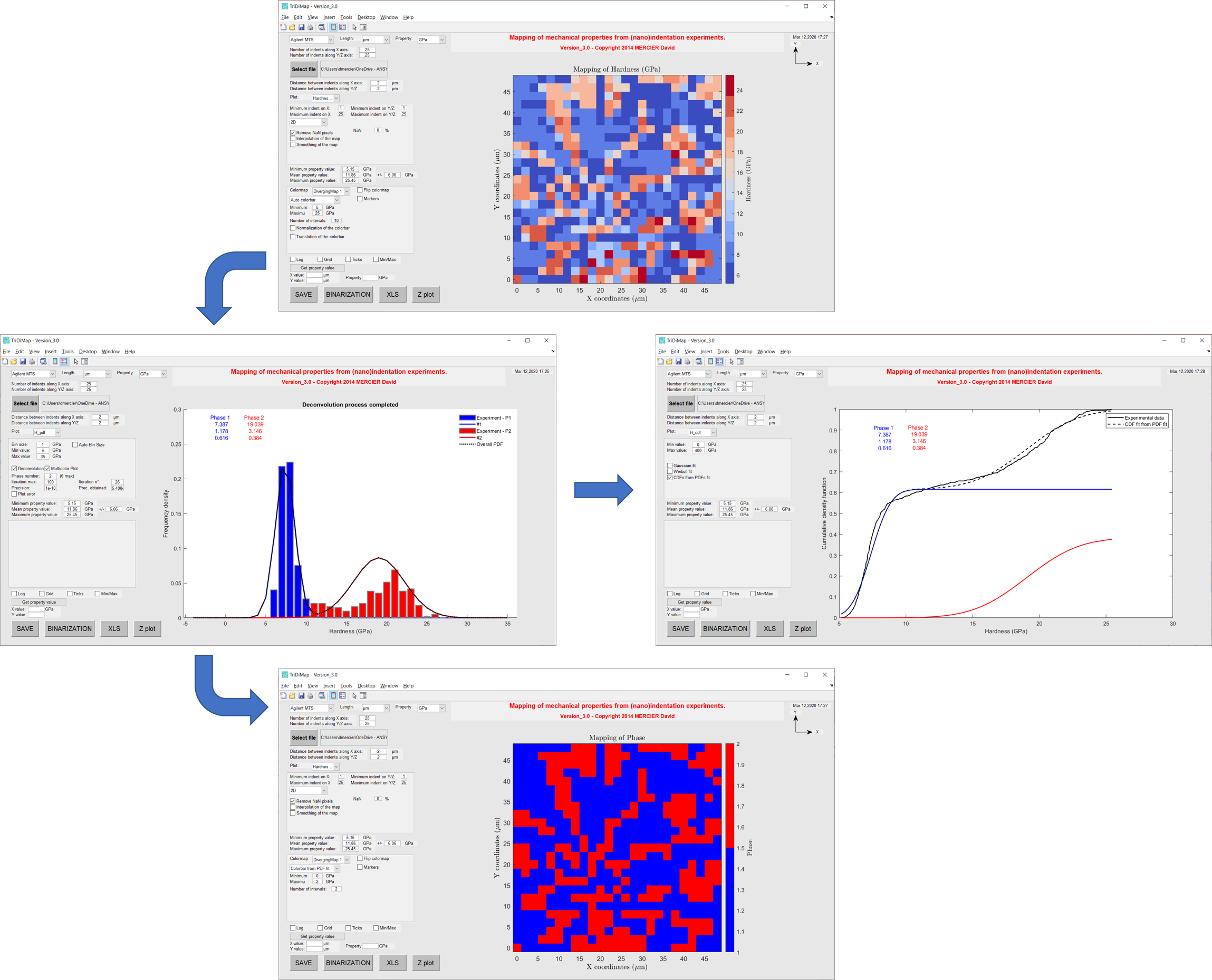Figure 28 Cumulative distribution of hardness valuesFigure 29 Cumulative distributions of hardness values with Weibull fit